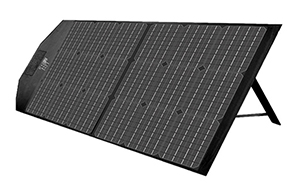# How to Calculate the Power Generation of a Solar Panel?

If you want to install a 100-300W solar panel, to calculate its power generation, you must first consider the following variables.

##1. Conversion efficiency of solar panels

Power (W) tells how much electricity the solar panel can produce, while efficiency tells how much sunlight the solar panel can convert into electricity. A simple example: If the efficiency of a solar panel is 15%, it means that the solar panel is able to convert 15% of sunlight into electricity. However, various factors can affect efficiency. The most common is the shaded area around the roof. If it is blocked by buildings or nearby trees, you can adjust the orientation of the solar panels and trim the branches of the trees. If there is a lot of dirt, contamination and dust. These are all harmful factors that can cause solar panel degradation over time, and you can clean your solar panels regularly. If it is rainy weather, this is inevitable.

2. Location (southern hemisphere, northern hemisphere, high latitude, low latitude)

The location determines how much electricity the solar panel can use. Solar power was first adopted in the sunny Southwest.

3. Installation direction of the solar panel

The direction the solar panel is facing also affects the amount of electricity produced. The recommended orientation for solar installations can be either south or west, depending on the region where your location is located.

4. Determine standard test conditions

Standard Test Conditions (STC), which are determined after testing solar panels in the laboratory. Standard test conditions for PV modules are 77 degrees Fahrenheit with 1000W of sunlight per square mile. If you achieve these perfect conditions, a solar panel rated at 250W will produce exactly 250W of electricity.

## Calculation of solar power generation

The formula for calculating the power generation of a solar panel is average sunshine duration × solar panel wattage × 75% = daily watt-hours. 75% accounts for all the above variables.

As an example: Let's say you live in a place with about 5 hours of average sunshine and the panels are rated at 200 watts. Calculate the power generation of the solar panel according to the above formula: 5hx200Wx0.75=750W/day.